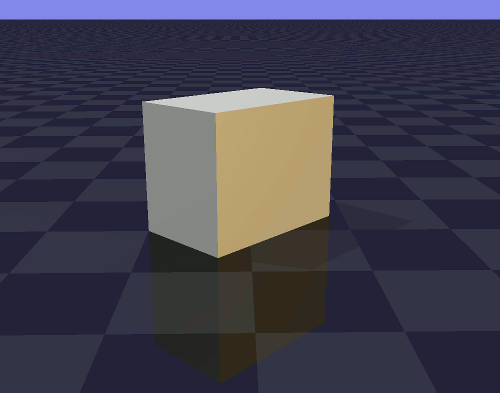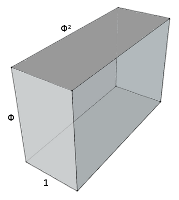# The other Golden Rectangle and Golden Cuboid

In mathematics the word “golden” is used for several shapes and forms that are based on the Golden Ratio, for instance one specific rectangle and one specific cuboid. But another rectangle and another cuboid are also based on the Golden Ratio.

### Golden Rectangle 2A (dynamic) rectangle with ratio 1 : square root of Phi and with diagonal Phi is not known as a “Golden Rectangle”.
It can be divided by Phi such that the major part is another golden rectangle and the minor part are two golden rectangles. This can be repeated ad infinitum (fractalic): every next rectangle is smaller and rotated 90 degrees.
Diagonal division makes a “Kepler triangle”, of which the edges are in geometrical progression, 1 : √Phi : Phi.
The Kepler triangle is sometimes called “golden triangle”, though there is another triangle that is more often called "golden triangle".
Mark Reynolds: “The square root of the golden section, root-phi, is at times not given its due in discussions regarding the golden section. This is an unfortunate situation because its geometric properties provide us with a unique and rarely applied design tool.”

### Golden Rectangle 1A (dynamic) rectangle with the ratio of 1 : Phi is known as “Golden Rectangle”.
It divides by Phi in a square (major) and itself in a quarter turn (minor).
It divides in a fractal way in combination with the square.
This 1 : Phi rectangle is often used as an aesthetical standard.

### Golden Cuboid 2Another rectangular cuboid, with ratios 1 : √Phi : Phi,
not known as a “Golden Cuboid”.
Two opposite faces are Phi rectangles (dark),
two opposite faces are big √Phi rectangles, and
two opposite faces are small √Phi rectangles.
The yellow rectangles have equal ratio 1 : √Phi = √Phi : Phi.
The diagonal (green) plane is a square.3D wiggle stereoin three different positions

### Golden Cuboid 1A cuboid with ratio 1 : Phi : Phi² is sometimes called a “Golden Cuboid”, e.g. listening room for audiophiles. Four opposite faces have ratio 1 : Phi, and two opposite faces have ratio 1 : Phi²

Jan Maris, august 2009

(august 2009 basic content. lay-out changes, small corrections and additions later)
Three well-known not-golden rectangles:1 : 1
square1 : 21 : √2
diagonal plane of a cube
a dynamic rectangle,
first described by
G. C. Lichtenberg
ISO 216 (A4 paper size)

Golden Ratio

A line divided by the “Golden Ratio”
1 : 0.5(sqrt(5)-1)+1
(= 1.61803399... = Φ = Phi)
has ratios 1 : major = major : minor.
Golden PyramidA pyramid of which the apothem is Phi times half the base width (Kepler tri­angle) is sometimes called a “golden pyramid”.